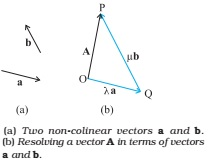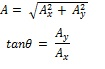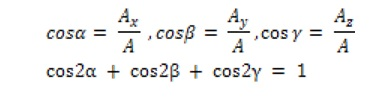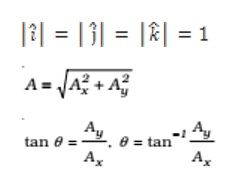## Resolution of a Vector in a Plane:

Let a and b be any two nonzero vectors in a plane with different directions and let A be another vector in the same plane. A can be expressed as a sum of two vectors – one obtained by multiplying a by a real number and the other obtained by multiplying b by another real number. To see this, let O and P be the tail and head of the vector A. Then, through O, draw a straight line parallel to a, and through P, a straight line parallel to b. Let them intersect at Q.  Then, we haveA = OP = OQ + QP

But since OQ is parallel to a, and QP is parallel to b, we can write:

OQ = λ a, and QP = μb

where λ and µ are real numbers.

Therefore, A = λ a + µ b

### Resolution of a Vector into Rectangular Components:

If any vector A subtends an angle θ with x-axis, then its

Horizontal component Ax = A cos θ
Vertical component Ay = A sin θMagnitude of vector### Direction Cosines of a Vector:

If any vector A subtend angles α, β and γ with x – axis, y – axis and z – axis respectively and its components along these axes are Ax, Ay and Az then## Unit Vector:

A unit vector is a vector of unit magnitude and points in a particular direction. It has no dimension and unit. It is used to specify a direction only. Unit vectors along the x-, y and z-axes of a rectangular coordinate system are denoted by  î, ĵ and  k  respectively,

Since these are unit vectors, we haveThese unit vectors are perpendicular to each other.